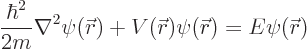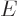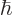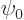### A.13 In­te­gral Schrö­din­ger equa­tion

The Hamil­ton­ian eigen­value prob­lem, or time-in­de­pen­dent Schrö­din­ger equa­tion, is the cen­tral equa­tion of quan­tum me­chan­ics. It readsHereis the wave func­tion,is the en­ergy of the state de­scribed by the wave func­tion,is the po­ten­tial en­ergy,is the mass of the par­ti­cle, andis the scaled Planck con­stant.

The equa­tion also in­volves the Lapla­cian op­er­a­tor, de­fined asThere­fore the Hamil­ton­ian eigen­value prob­lem in­volves par­tial de­riv­a­tives, and it is called a par­tial dif­fer­en­tial equa­tion.

How­ever, it is pos­si­ble to ma­nip­u­late the equa­tion so that the wave func­tionap­pears in­side an in­te­gral rather than in­side par­tial de­riv­a­tives. The equa­tion that you get this way is called the in­te­gral Schrö­din­ger equa­tion. It takes the form, {D.31}:(A.42)

Hereis any wave func­tion of en­ergyin free space. In other wordsis any wave func­tion for the par­ti­cle in the ab­sence of the po­ten­tial. The con­stantis a mea­sure of the en­ergy of the par­ti­cle. It also cor­re­sponds to a wave num­ber far from the po­ten­tial. While not strictly re­quired, the in­te­gral Schrö­din­ger equa­tion above tends to be most suited for par­ti­cles in in­fi­nite space.